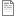# ESM_SIM_DATA_ANN_UNPRUNNED

ESM_sim_data_3. Estimates of contribution of MCSim parameters to variation in biodiversity metrics based on contribution statistics from artificial neural networks (ANNs) trained and validated on simulated metacommunity outcomes.

## File:ESM_SIM_DATA_ANN_UNPRUNNED.csv (28.45 KB)
Variables
Diversity Metric ID
• Label:
• Definition: Diversity Metric ID
• Type: Nominal
• Missing values: None specified
Predictor Variable
• Label:
• Definition: Predictor Variable
• Type: Nominal
• Missing values: None specified
Predictor contribution to variation in response variable
• Label:
• Definition: Predictor contribution to variation in response variable
• Type: Nominal
• Missing values: None specified
Contribution p value
• Label:
• Definition: Contribution p value
• Type: Nominal
• Missing values: None specified
Predictor relative importance (RI) to variatino in response variable
• Label:
• Definition: Predictor relative importance (RI) to variatino in response variable
• Type: Nominal
• Missing values: None specified
RI p value
• Label:
• Definition: RI p value
• Type: Nominal
• Missing values: None specified
Keep predictor in pruned model?
• Label:
• Definition: Keep predictor in pruned model?
• Type: Nominal
• Missing values: None specified
N permutations used to estimate p values
• Label:
• Definition: N permutations used to estimate p values
• Type: Nominal
• Missing values: None specified
Data Source Details